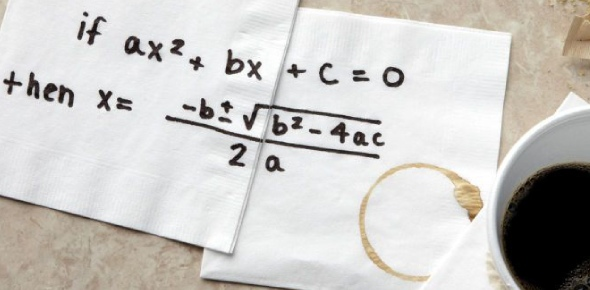What will be the mean, median, and mode of the following sample? - ProProfs DiscussTopicsMoreProductsMore+ Ask Question

# What will be the mean, median, and mode of the following sample?4, 5, 6, 1, 1, 2, 5, 7, 5Change Image    Delete

A. 4, 4, 5
B. 3, 5, 4
C. 4, 3, 5
D. 4, 5, 5

This question is part of Six Sigma Green Belt Exam
Asked by PracticeExcellence, Last updated: Jul 09, 2020

###Request 0FollowShareAnswer AnonymouslyAnswer LaterCopy Link#### M. Krasinski

M. Krasinski, Content Writer, Columbus

Based on the given, the answer to this question is D. 4,5,5. In order to compute the mean, all of the data should be added together. The sum will then be divided by the total number of figures that are given. The median will require you to put all of the figures in order. Based on the numbers, find the middle value.

The mode is the number which will appear most in the given data. Let us use the given above to test the answer. To find the mean, you can add the following: 4+5+6+1+1+2+5+7+5 =36/9 =4. This means that the mean is 4. For median, it will be 1, 1, 2, 4, (5,) 5, 5, 6, 7 =5. For the mode, the answer is also 5 as it appears 3 times in the given.#### John Smith

John Smith4,5,5Search for Google imagesSelect a recommended image
Upload from your computerCancelSearch for Google imagesSelect a recommended image
Upload from your computerCancelSearch for Google imagesSelect a recommended image
Upload from your computerCancel